# Introduction to R - 4th lesson (graphics)

Nathalie Villa-Vialaneix - http://www.nathalievilla.org
September 14-16th, 2015

Master TIDE, Université Paris 1## Introduction• basic concepts
• graphics parameters
• graphics devices

### Basic concepts on making a plot

• When making a plot, the user must first choose to what device the plot is sent (a new window, a png file, a postscript file,…) and set the graphic parameters (with `par`)
• A plot is made in several steps:
1. first, a high level graphic function is called (`plot`, `boxplot`, `pie`…) that initiates the graphic;
2. then, one or several (or even none) low level graphic functions are called (`legend`, `points`, `lines`…) to add elements to the graphic.

Eventually, the graphic can be exported in a file (png, postscript, pdf, …) after.

### Graphics parameters

``````data(airquality)
par(bg="lightyellow", mfcol=c(1,2), pch="+")
plot(airquality\$Ozone)
plot(factor(airquality\$Month))
``````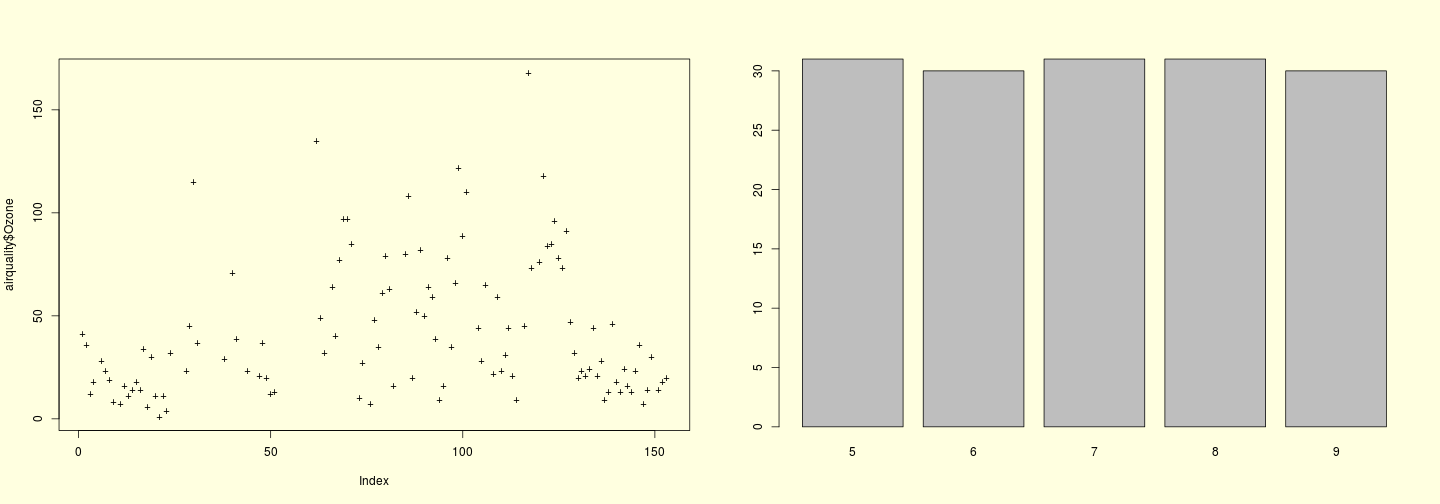### Graphics parameters

Important graphics parameters include:

• `pch`: the plotting symbol (default is an open circle)
• `lty`: the line type (default is a solide line)
• `lwd`: the line width, specified as an integer multiple
• `col`: the plotting color (default is black)
• `las`: the orientation of the axis label on the plot (default is 0, which corresponds to labels parallel to the axes, 1 is for horizontal labels, 2 for labels perpendicular to the axes and 3 to vertical labels)
• `bg`: the background color (default: white)

### Graphics parameters

Important graphics parameters include:

• `mar`: the margin size (default is `c(5,4,4,2)`, bottom, left, top, right)
• `oma`: the outer margin size (default is 0)
• `mfrow`: number of plots per row, column (plots are filled row-wise)
• `mfcol`: number of plots per row, column (plots are filled col-wise)

### Graphics device

By default graphics are shown on screen but this can be changed using a specific command like `png`, `svg`, `pdf`… Available graphics devices can be found using `?Device`.

``````png("myFistGraphic.png", width=600,
height=600)
par(bg="transparent", lty=2, lwd=2,
col="darkred")
plot(airquality\$Wind, airquality\$Ozone)
dev.off() # close the graphics device
``````

### Exporting a graphic

A graphics made on the screen can be exported in a graphic device (but this is not an exact operation)

``````par(bg="transparent", lty=2, lwd=2,
mar=rep(2,4), col="darkred")
plot(airquality\$Wind, airquality\$Ozone)
``````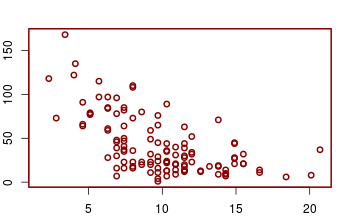``````dev.print(png, file="mySecondGraphic.png",
width=600, height=600)
``````
``````png
2
``````

## Generic function plot• numeric/non numeric variables
• one or two variables

### plot for one numeric variable

This function displays the value of the variable versus its index, as a scatterplot (default) or a line (`type="l"`):

``````plot(airquality\$Ozone, type="l")
``````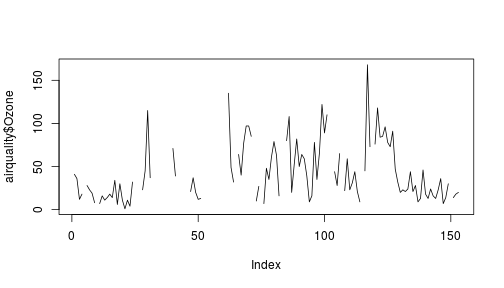… meaningful only for e.g., time series.

### plot for one numeric variable

The standard graphics paramaters can be passed to the function (see also argument `type` in help):

``````plot(airquality\$Ozone, main="Ozone",
type="b", pch=19, col="darkred")
``````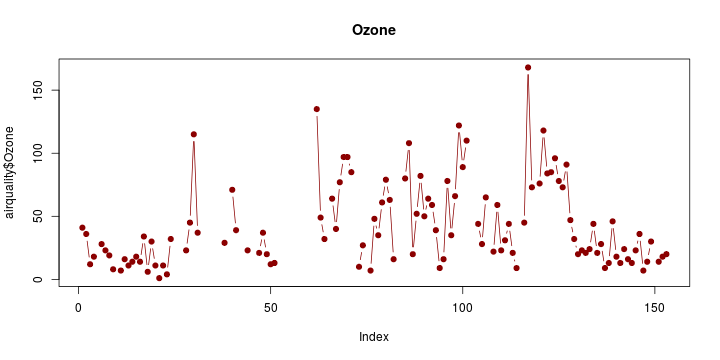### plot for one non numeric variable

This function displays the barplot of the distribution of the variable:

``````plot(factor(airquality\$Month), main="Month",
col="pink", lwd=2, las=3,
names=c("May","June","July","Aug.","Sept"))
``````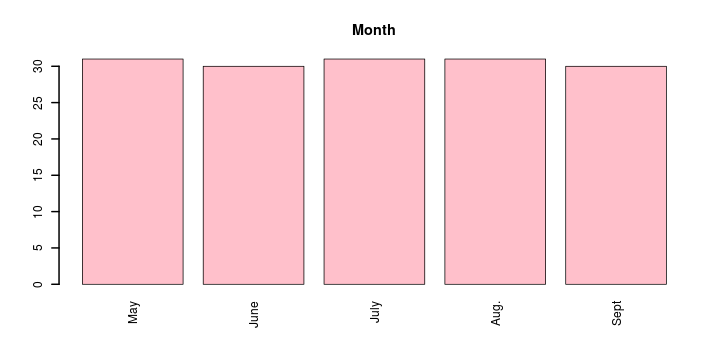### plot for two numeric variables

This function displays the value of the first variable versus the value of the second variable.

``````par(mfrow=c(1,2))
plot(airquality\$Wind, airquality\$Ozone)
plot(airquality\$Ozone~airquality\$Wind)
``````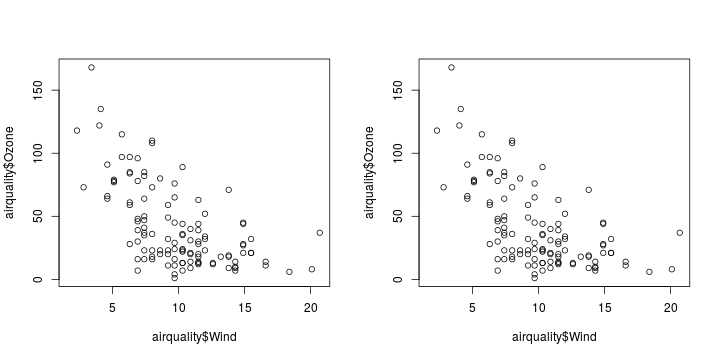### plot for two variables: one numeric and one non numeric

This function has different behaviors depending on its syntax:

``````par(mfrow=c(1,2))
plot(airquality[,3], factor(airquality[,5]))
plot(factor(airquality[,5]), airquality[,3])
``````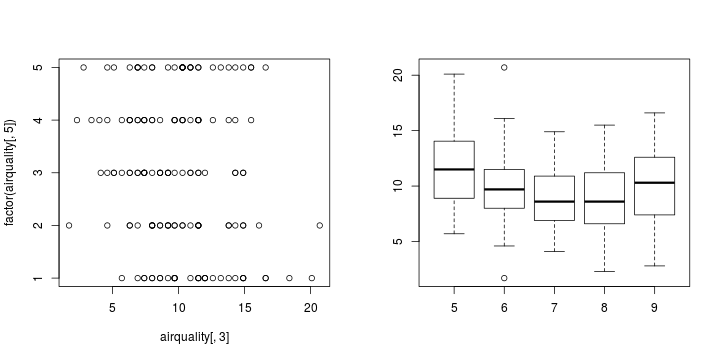### plot for two variables: one numeric and one non numeric

``````par(mfrow=c(1,2))
plot(airquality[,3]~factor(airquality[,5]))
plot(factor(airquality[,5])~airquality[,3],
col=rainbow(nlevels(factor(airquality[,5]))))
``````